Function Repository Resource:

# PolynomialApproximation

Approximate a function with a polynomial

Contributed by: Sander Huisman
 ResourceFunction["PolynomialApproximation"][f,{x,xmin,xmax},n, "type"] finds an nth order polynomial of a given type that approximates the function f for x∈[xmin,xmax].

## Details

Supported values of "type" include:
 "Taylor" Taylor expansion around (xmin+xmax)/2 "Equispaced" Polynomial going through n+1 points equispaced between xmin and xmax "Chebyshev" Polynomial going through n+1 Chebyshev nodes "Remez" Iteratively optimize the nodes starting with Chebyshev nodes {“Remez”, m} Remez with m iterations

## Examples

### Basic Examples (5)

Find the second-order Taylor polynomial approximating the sine function:

 In:=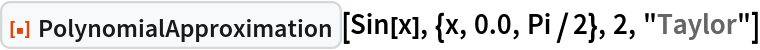Out=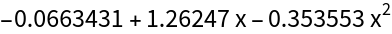Compare with the original function:

 In:=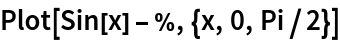Out=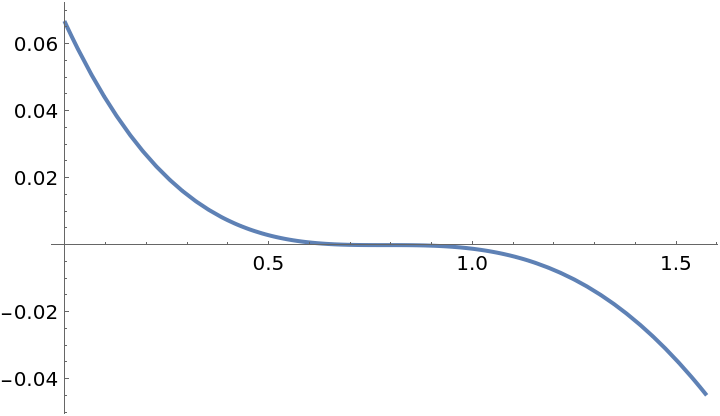Use equispaced points to get a better approximation:

 In:=Out=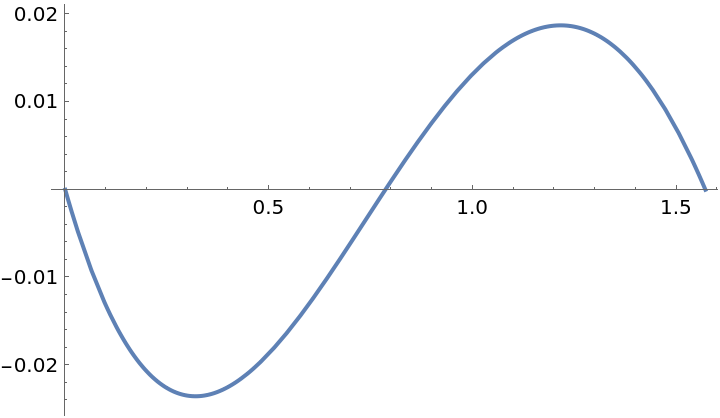Use Chebyshev points to get an even better approximation:

 In:=Out=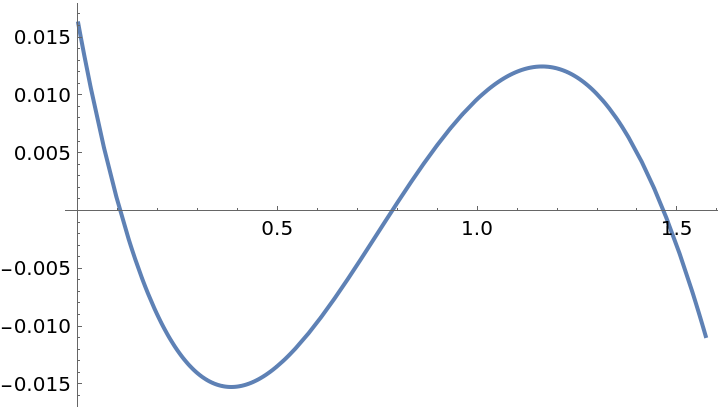The polynomial produced by the Remez method yields a nearly-optimal approximation:

 In:=Out=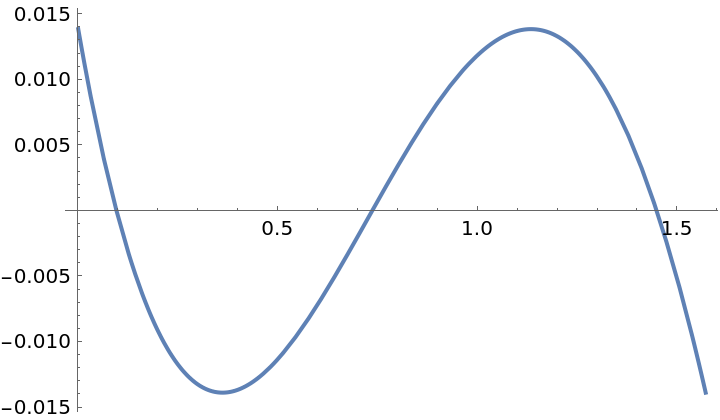### Scope (1)

Perform 6 iterations for the Remez method, and then compare the error:

 In:=Out=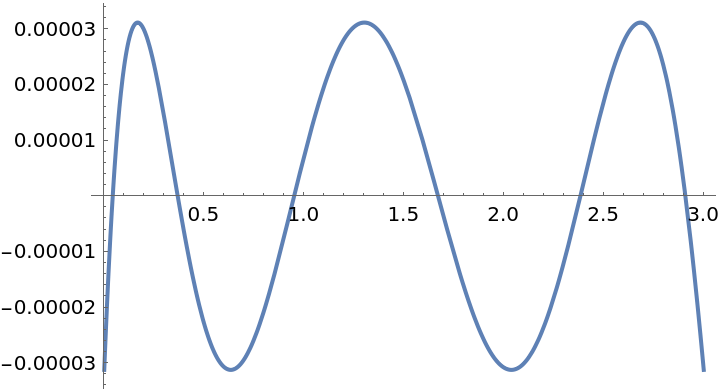### Applications (2)

Find a function that can approximate the cosine function for angles up to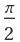:

 In:=Out=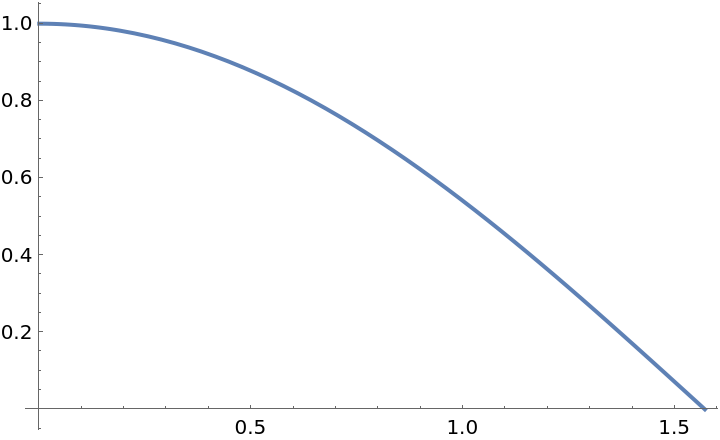Calculate several orders and compare the maximum absolute error:

 In:=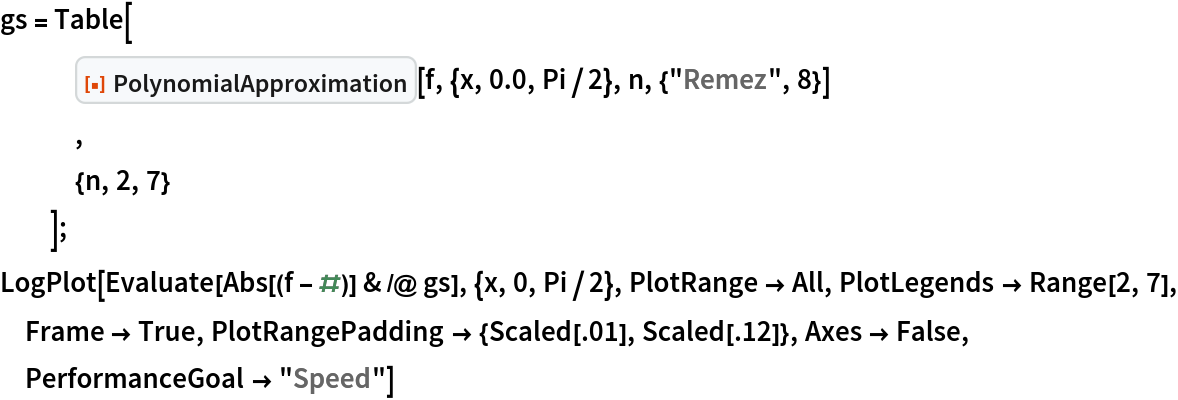Out=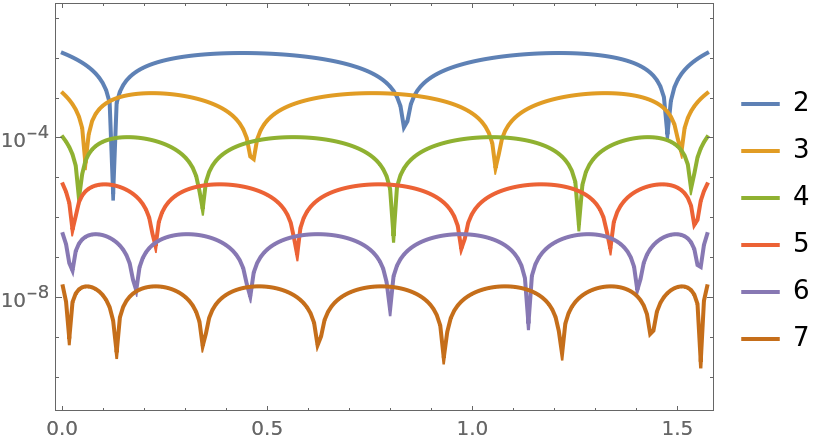### Possible Issues (4)

The Remez method does not always converge:

 In:=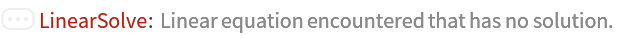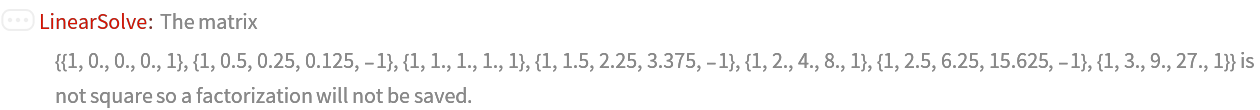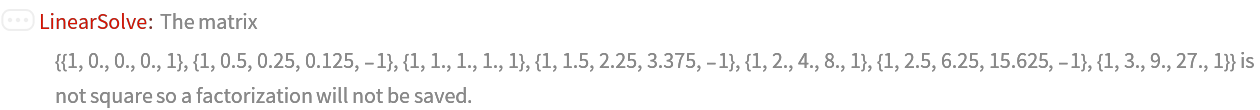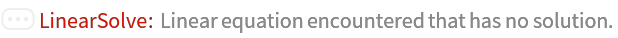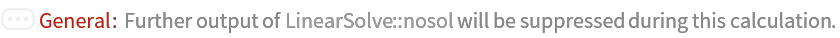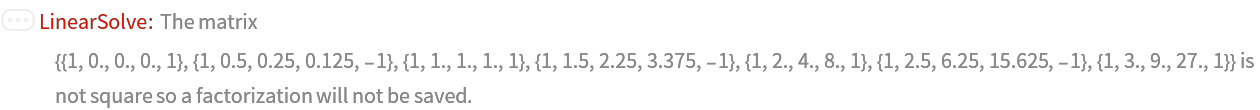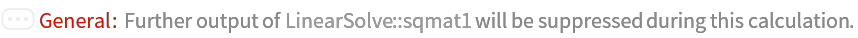Out=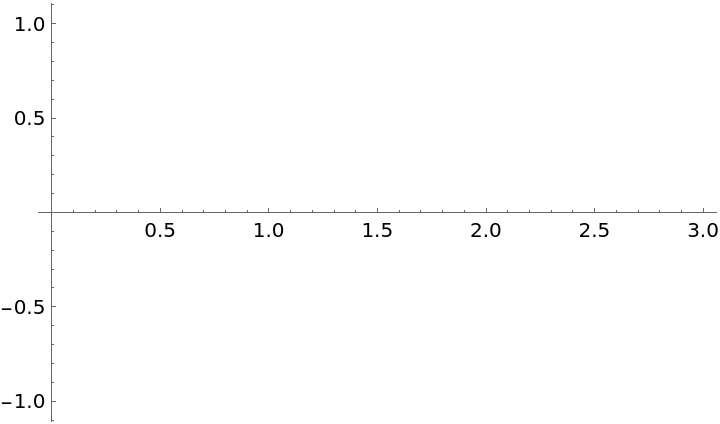Higher order Remez might not converge to a good solution:

 In:=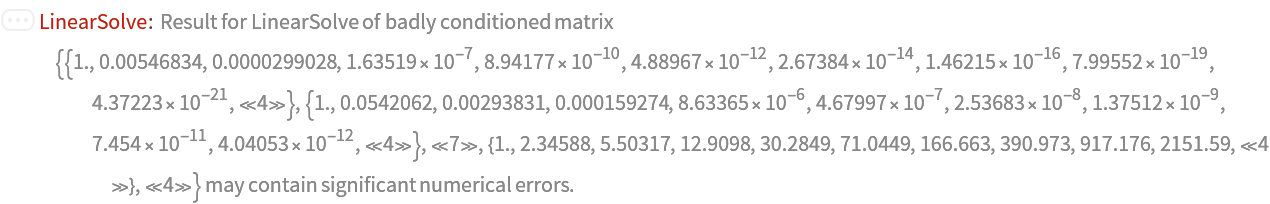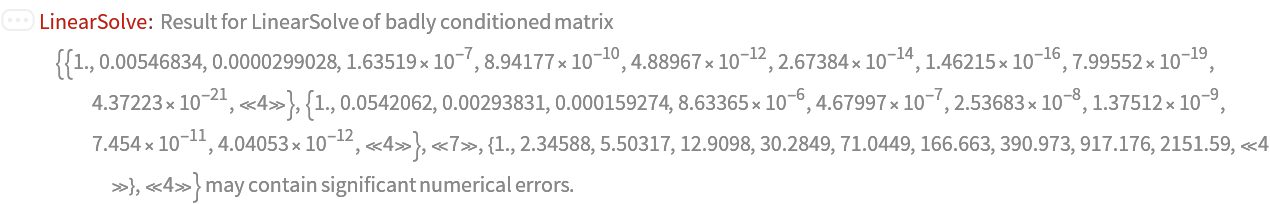Out=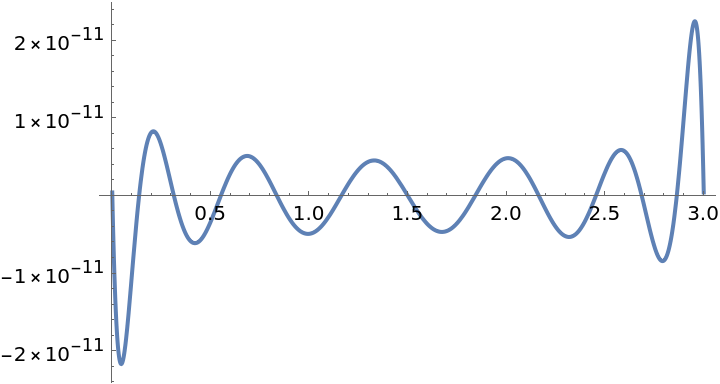Higher order Remez might be prone to numerical errors:

 In:=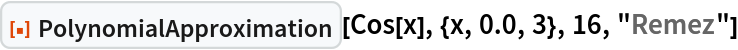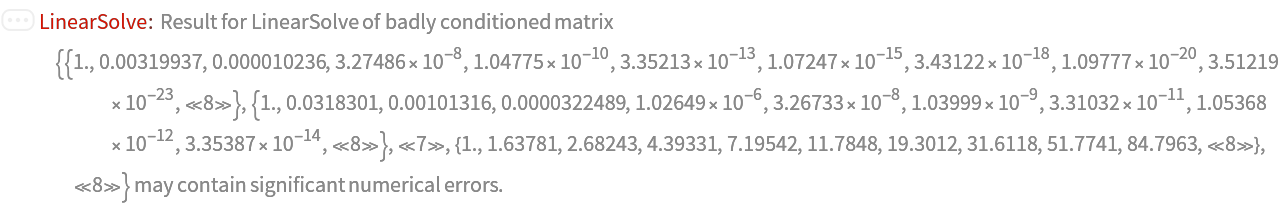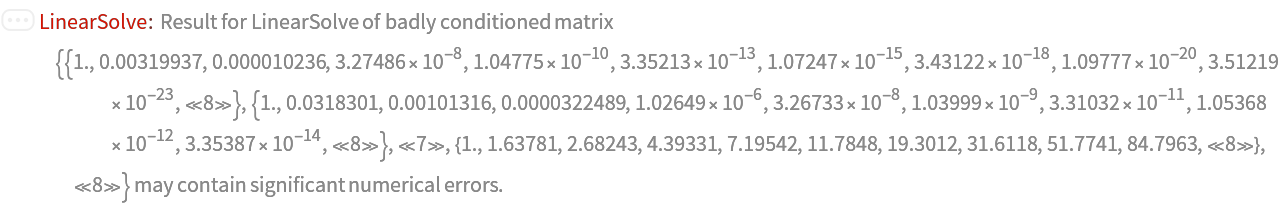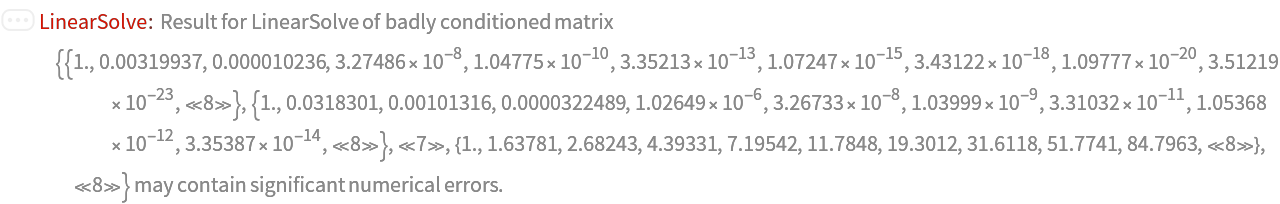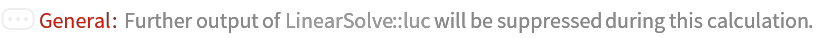Out=Functions that are not continuous can not be approximated very well:

 In:=Out=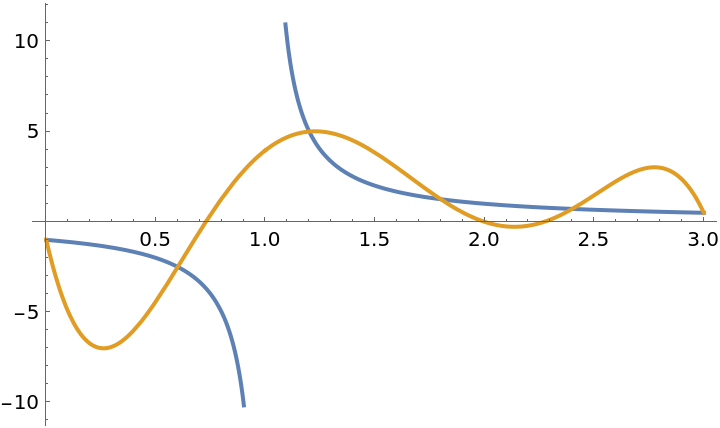### Neat Examples (3)

Find a function that approximates a normal distribution:

 In:=Out=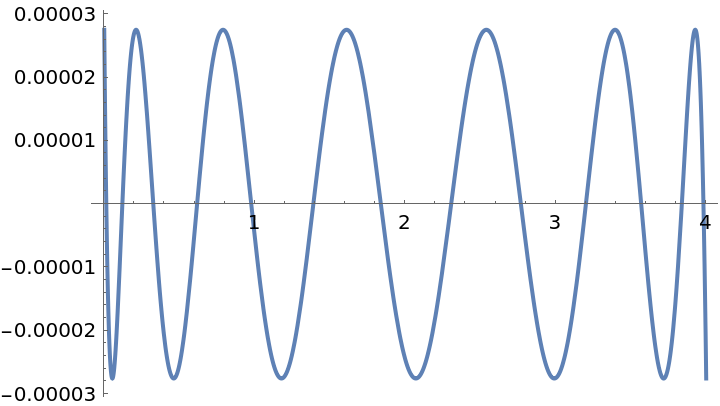Find the maximum error:

 In:=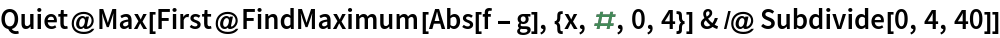Out=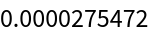Compare that to the Chebyshev case:

 In:=Out=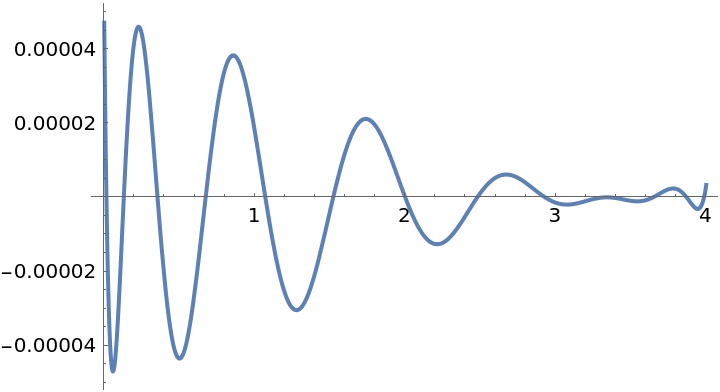In:=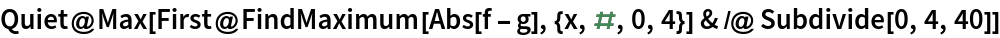Out=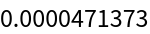SHuisman

## Requirements

Wolfram Language 13.2 (December 2022) or above

## Version History

• 1.0.0 – 11 August 2023